#### How to Use Rand Function in Excel

In this lesson, you can learn how to use RAND function in Excel. The RAND is a function that would return with an equally distributed random number that is either greater than or equal to 0 and less than 1. The new random real number will be returned every time that it is being calculated.

RAND function draws a value between 0 and 1. The Syntax is RAND(), and there are no arguments for it.

## Rand function syntax

=RAND()

Since the operation is done from scratch each time per sheet, it is best to immediately convert it into value. Otherwise it draws a new value each time you change the data in the file. Often the result of this function is multiplied by or divided to obtain an order of magnitude of numbers that we are interested in

• =RAND()*1000
• =0.5+RAND()/10
• =RAND()*(100-50)+50
• =RAND()*(A1-A2)+A2
• =INT(RAND()*(A1-A2)+A2)

The function is sometimes used most often to create a variety of data, which look realistic. Most of the data in Best Excel Tutorial was created using this function.

## Examples of Rand function

### Example 1: Standard RAND Formula

We would like to know the rand, which is why we are using the RAND function.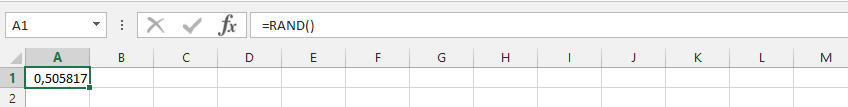=RAND()

### Example 2: Numbers and RAND

This is for finding out the even numbers with the rand function.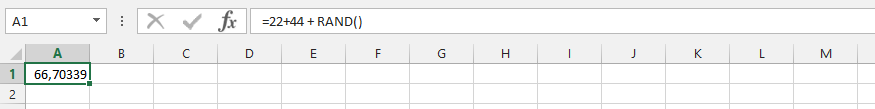=22+44+RAND()

### Example 3: Evenly 100

This is for finding out the even numbers for 100, with the rand function.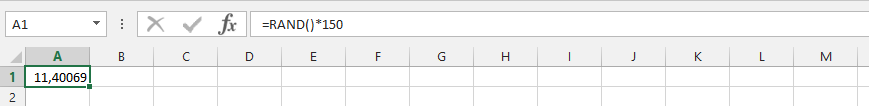=RAND() *150

### Example 4: RAND Function with Flexibilities

This is to try and find out the rand, since we have already layout different data.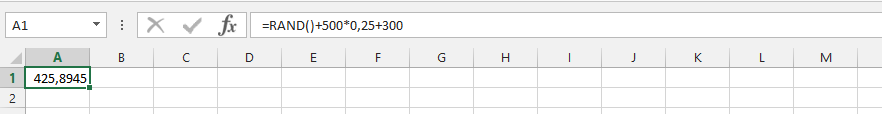=RAND() +500*0.25+300

### Example 5: SUMPRODUCT and RAND

We’d have different numbers that have been layout, and would like to know what it would mean for finding answers.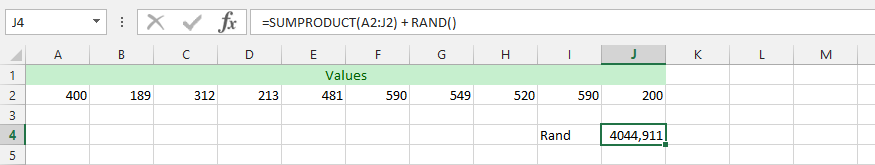=SUMPRODUCT(A2:J2)+RAND()

### Example 6: SUM and RAND

The data is layout, and we would like to acknowledge the randomly generated number. This is to find out the even numbers.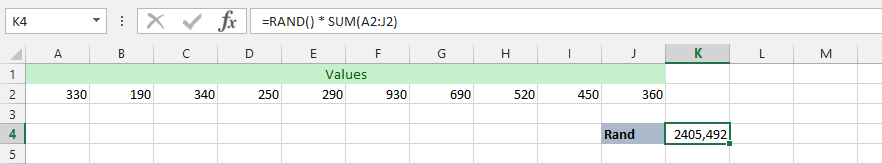=RAND() *SUM(A2:J2)

### Example 7: Double SUM and RAND

The data has been spread, and we would like to find out what the even numbers will be, and subtract 15 percent from the total number, in order to get the evenly generated number.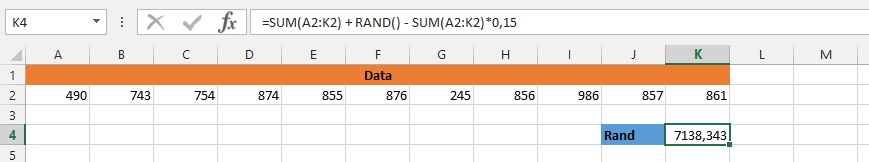=SUM(A2:K2)+RAND()-SUM(A2:K2)*0.15

### Example 8: Double SUM and Double RAND

The circumstances is we are examining how it would look for the even numbers when we are trying to find the answers that correspond with the data.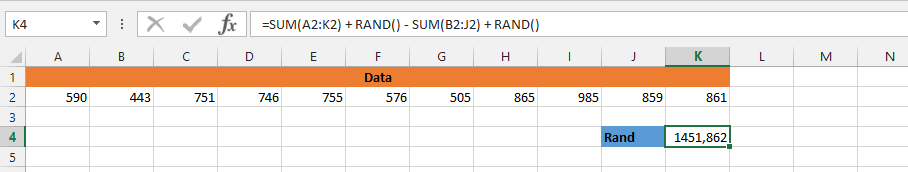=SUM(A2:K2)+RAND()-SUM(B2:J2)+RAND()

### Example 9: AVERAGE and RAND

We are using the previous data to find the answer, with the average at its center. This is why we are using both AVERAGE and RAND formulas.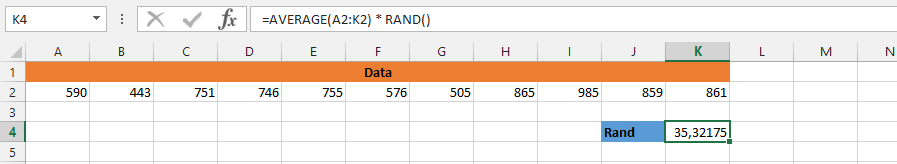=AVERAGE(A2:K2)*RAND()

### Example 10: Rand and Average

This time we’d have no data, but we have to find out the random and average functions working together to find the answers.

See also  How to use Vlookup to find a Value Greater Than a Certain Number in Excel?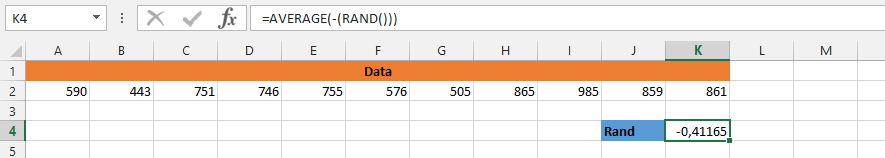=AVERAGE(-(RAND())))